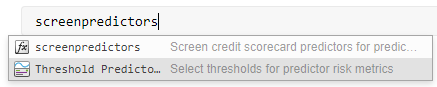Threshold Predictors

Select thresholds for predictor risk metrics in the Live Editor

Description

The Threshold Predictors task lets you interactively set credit scorecard predictor thresholds for one or more risk metrics computed for a set of predictors, or features. Risk metric thresholds are part of the feature selection process before building a credit scorecard. The task automatically generates MATLAB® code for your live script.

• Select risk metrics from the columns of a table of risk metric data.

• Specify thresholds for the risk metrics, separating the rows of predictors into color-coded Pass, Fail, or Undecided regions.

• Visualize the labeled and color-coded risk metric values for each thresholded metric.

To add the Threshold Predictors task to a live script in the MATLAB Editor:

• On the Live Editor tab, select Task > Threshold Predictors.• In a code block in the script, type a relevant keyword, such as screenpredictors. Select Threshold Predictors from the suggested command completions.Parameters

The Predictor metrics table must be a numeric MATLAB table. The columns of the Predictor metrics table contain the values for a particular risk metric (for example, information value or accuracy ratio) for a set of model predictors. The rows of the table contain the values of each risk metric for a particular predictor. The Predictor metrics table must have defined row names.

Typically, you create the Predictor metrics table using the screenpredictors function. screenpredictors takes a creditscorecard input data set and calculates the risk metrics table.

Example: metric_table = screenpredictors(data,'IDVar',idvar,'ResponseVar',responsevar)

The Select thresholded metrics list shows which metrics have thresholds specified. The Select thresholded metrics drop-down box contains the risk metrics defined in the columns of the Predictor metrics table.

To specify a threshold:

1. Select a risk metric from the Select thresholded metrics drop-down box and click the plusbutton. The metric is added to the Select thresholded metrics list box and the Predictors plot displays with a default threshold and region labels.

2. To adjust a threshold, drag the associated threshold line or use the Thresholds and Labels spinner controls.

3. Set additional thresholds by clicking the Predictors plot at the desired value.

4. Select a different metric from the Select thresholded metrics list box. The Predictors plot updates to show the associated metric bar chart with its overlayed threshold lines.

To remove a threshold:

• Select the threshold line or the associated Thresholds and Labels spinner and click the line deletebutton on the Predictors plot. You can remove all thresholds for the selected risk metric by clicking the minusbutton next to the Select thresholded metrics list box.

When using a Predictor metrics table that is created using the screenpredictors function, you can set thresholds for any of the following metrics:

• InfoValue

• Entropy

• Accuracy Ratio

• AUROC

• Gini

• Chi2PValue

• PercentMissing

The Thresholds and Labels controls are composed of spinners for each specified threshold of the currently selected risk metric and drop-down boxes that set the labels for the surrounding regions to Pass, Fail, or Undecided.

The Thresholds and Labels spinners are sorted in descending order from top to bottom. The region labels can be set to Pass, Fail, or Undecided where the region label defines the label for all metric values that lie on a particular side of a threshold.

Check the Display label table check box to display the current set of labeled metric values. The label table contains the columns from the Predictor metrics table for which there are specified thresholds. The entries in the label table are categorical labels (Pass, Fail, or Undecided) based on which region each metric value is found.

Tips

• To sort the predictors in the Predictors plot, click .To revert to the original sort order, click .

• Each time you add a new threshold by clicking the Predictors plot, a new set of controls is added to the Thresholds and Labels section. Use the spinner to fine tune the threshold value. Use the label drop-down box to set the appropriate label (Pass, Fail, or Undecided) for the newly defined region of metric values.